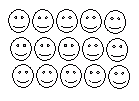Name: ___________________Date:___________________

 Method: Step 1: Count the pictures in each row. Step 2: Multiply the number of patterns in each row by the total number of rows. Example: Use the figure to find 3 times 5.This is made of 3 rows of 5 in each, we hence write as 5*3. Answer: 15 Directions: Answer the following questions also write at least ten examples of your own.